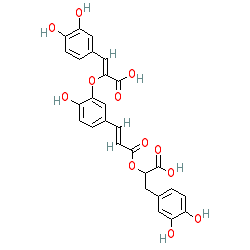=====================================================SMILES: OC(C(OC(/C=C/C1=CC=C(O)C(O/C(C(O)=O)=C\C2=CC(O)=C(O)C=C2)=C1)=O)CC3=CC=C(O)C(O)=C3)=O
InChIKey: HFTLCJIFEZUOCR-JKXXRSRDSA-N

–EOF–